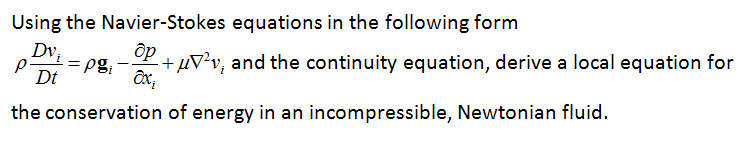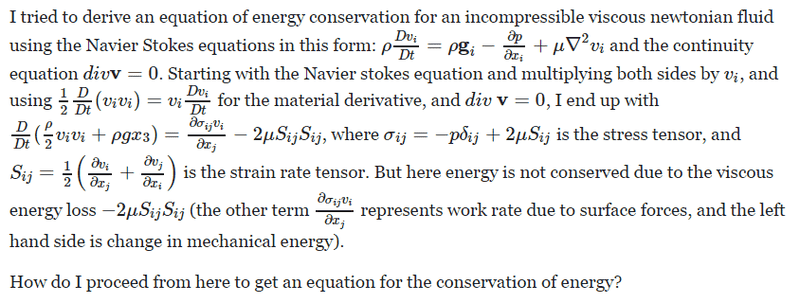# Energy conservation for a Newtonian fluid?

## Homework Statementρ= density, vi = i-th velocity component, gi=i-th component of gravity vector, p=pressure, μ= viscosity, D/Dt = material derivative

## Homework Equations

Continuity equation: div v = 0

## The Attempt at a SolutionChestermiller
Mentor

## Homework Statement

View attachment 203392
ρ= density, vi = i-th velocity component, gi=i-th component of gravity vector, p=pressure, μ= viscosity, D/Dt = material derivative

## Homework Equations

Continuity equation: div v = 0

## The Attempt at a Solution

View attachment 203393
The derivation you are looking for is in Transport Phenomena by Bird, Stewart, and Lightfoot. It involves dotting the equation of motion (momentum balance equation) with the velocity vector. Incidentally, this is called the Mechanical Energy Balance Equation. It can be combined with the Overall Energy Balance equation to yield the Thermal Energy Balance equation.